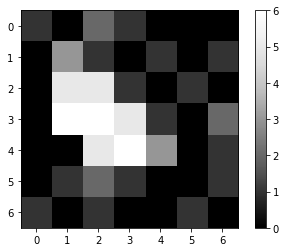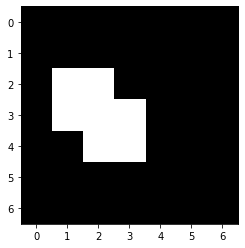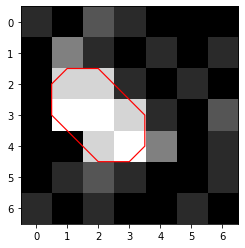# Image segmentation#

Image segmentation is the process of separating an image into multiple regions.

Let’s start again by defining an image as a two dimensional array and visualize it using pyclesperanto.

import numpy as np
from pyclesperanto_prototype import imshow
import matplotlib.pyplot as plt

image = np.asarray([
[1, 0, 2, 1, 0, 0, 0],
[0, 3, 1, 0, 1, 0, 1],
[0, 5, 5, 1, 0, 1, 0],
[0, 6, 6, 5, 1, 0, 2],
[0, 0, 5, 6, 3, 0, 1],
[0, 1, 2, 1, 0, 0, 1],
[1, 0, 1, 0, 0, 1, 0]
])

imshow(image, colorbar=True)## Binary images#

The most basic way of that is binarization, turning the image into a “positive” and a “negative” region. Typically, binary images are used for that, which could for example contain two different pixel values True and False representing “positive” and “negative”, respectively. Technically, every image can be interpreted as a binary image using the rationale “Every pixel is considered positive that is neither False nor 0.”

## Image thresholding#

A very basic algorithm for separating low intensity regions from high intensity regions in the image is thresholding. We will now make a new image containing True and False as pixel values depending on if the original image had intensity lower or higher a given threshold. As this image has just two different pixel values, it is a binary image:

threshold = 4

binary_image = image > threshold

binary_image

array([[False, False, False, False, False, False, False],
[False, False, False, False, False, False, False],
[False,  True,  True, False, False, False, False],
[False,  True,  True,  True, False, False, False],
[False, False,  True,  True, False, False, False],
[False, False, False, False, False, False, False],
[False, False, False, False, False, False, False]])

imshow(binary_image)Matplotlib might be more flexible when visualizing images, e.g. for drawing outlines around regions of interest:

# create a new plot
fig, axes = plt.subplots(1,1)


<matplotlib.contour.QuadContourSet at 0x1a542ce37f0>